# 数据结构之算法时间复杂度

## 数据结构之算法时间复杂度

• 找出算法中的基本语句

算法中执行次数最多的那条语句就是基本语句，通常是最内层循环的循环体。

• 计算基本语句的执行次数的数量级

只需计算基本语句执行次数的数量级，这就意味着只要保证基本语句执行次数的函数中的最高次幂正确即可，可以忽略所有低次幂和最高次幂的系数。这样能够简化算法分析，并且使注意力集中在最重要的一点上：增长率。

• 用大Ο记号表示算法的时间性能

将基本语句执行次数的数量级放入大Ο记号中。

### 如何推导大o阶呢？我们给出了下面 的推导方法：

1.用常数1取代运行时间中的所有加法常数。

2.在修改后的运行次数函数中，只保留最髙阶项。

3.如果最高阶项存在且不是1,则去除与这个项相乘的常数。

#include "stdio.h"

int main()
{
int i, j, x = 0, sum = 0, n = 100;  /* 执行1次 */
for( i = 1; i <= n; i++)    /* 执行n+1次 */
{
sum = sum + i;               /* 执行n次 */
for( j = 1; j <= n; j++)    /* 执行n*(n+1)次 */
{
x++;                /* 执行n*n次 */
sum = sum + x;      /* 执行n*n次 */
}
}
printf("%d", sum);          /* 执行1次 */
}

（直接相加的话,应该是T(n) = 1 + n+1 + n + n*(n+1) + n*n + n*n + 1 = 3n^2 + 3n + 3。现在用常数 1 取代运行时间中的所有加法常数，就是把T(n) = 3n^2 + 3n + 3中的最后一个3改为1. 就得到了 T(n) = 3n^2 + 3n + 1）

O(1) 常数阶 < O(logn) 对数阶 < O(n) 线性阶 < O(nlogn) < O(n^2) 平方阶 < O(n^3) < { O(2^n) < O(n!) < O(n^n) }

#include "stdio.h"

int main()
{
int i, sum = 0, n = 100;    /* 执行1次 */
for( i = 1; i <= n; i++)    /* 执行 n+1 次 */
{
sum = sum + i;          /* 执行n次 */
//printf("%d \n", sum);
}
printf("%d", sum);          /* 执行1次 */
}

    void MatrixMultiply(int A[n][n]，int B [n][n]，int C[n][n])

{

(1) for(int i=0; i <n; i++)                       //n+1

{

(2)      for (j=0;j < n; j++)                       //n*(n+1)

{

(3)           C[i][j]=0;                                  //n^2

(4)           for (k=0; k<n; k++)                 //n^2*(n+1)

{

(5)              C[i][j]=C[i][j]+A[i][k]*B[k][j]; //n^3

}

}

}

}


T(n) = 2n^3+3n^2+2n+1; 利用大O表示法,该算法的时间复杂度为O(n^3)。


void test_(int n)
{
i = 1, k = 100;
while (i<n)
{
k = k + 1;
i += 2;
}
}


void test_2(int b[]， int n)
{
int i, j, k;
for (i=0; i<n-1; i++)
{
k = i;
for (j=i+1; j<n; j++)
{
if (b[k] > b[j])
{
k = j;
}
}
x = b[i];
b[i] = b[k];
b[k] = x;
}
}

if (b[k] > b[j])
{
k = j;
}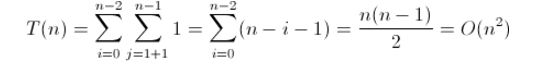void test_3(int n)
{
int i = 0, s = 0;
while (s<n)
{
i++;
s = s + i;
}
}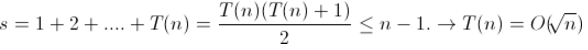void hanoi(int n, char a, char b, char c)
{
if (n==1)
{
printf("move %d disk from %c to %c \n", n, a, c);  //执行一次
}
else
{
hanoi(n-1, a, c, b);    //递归n-1次
printf("move %d disk from %c to %c \n", n, a, c);  //执行一次
hanoi(n-1, b, a, c);    //递归n-1次
}
}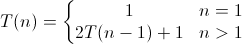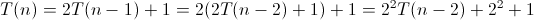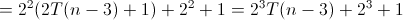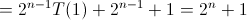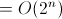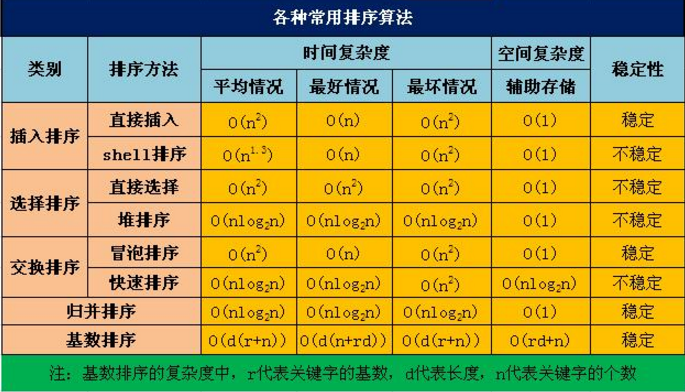08-304464
10-072万+
09-06463
11-282181
11-19356
02-221116
10-26
03-31
10-26794
11-241527
11-291万+
11-281890
08-27263

### “相关推荐”对你有帮助么？

•非常没帮助
•没帮助
•一般
•有帮助
•非常有帮助被折叠的  条评论 为什么被折叠?到【灌水乐园】发言¥1 ¥2 ¥4 ¥6 ¥10 ¥20获取中扫码支付点击重新获取扫码支付1.余额是钱包充值的虚拟货币，按照1:1的比例进行支付金额的抵扣。
2.余额无法直接购买下载，可以购买VIP、付费专栏及课程。余额充值